Ex 3.5

Chapter 3 Class 10 Pair of Linear Equations in Two Variables
Serial order wise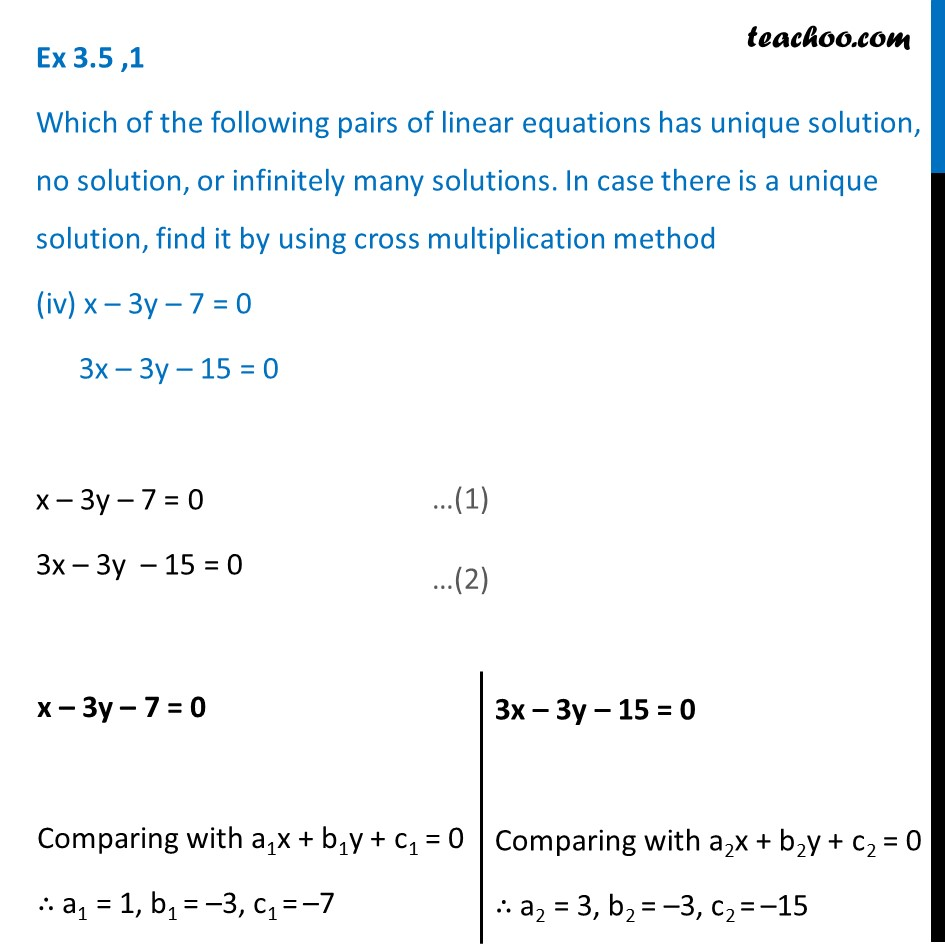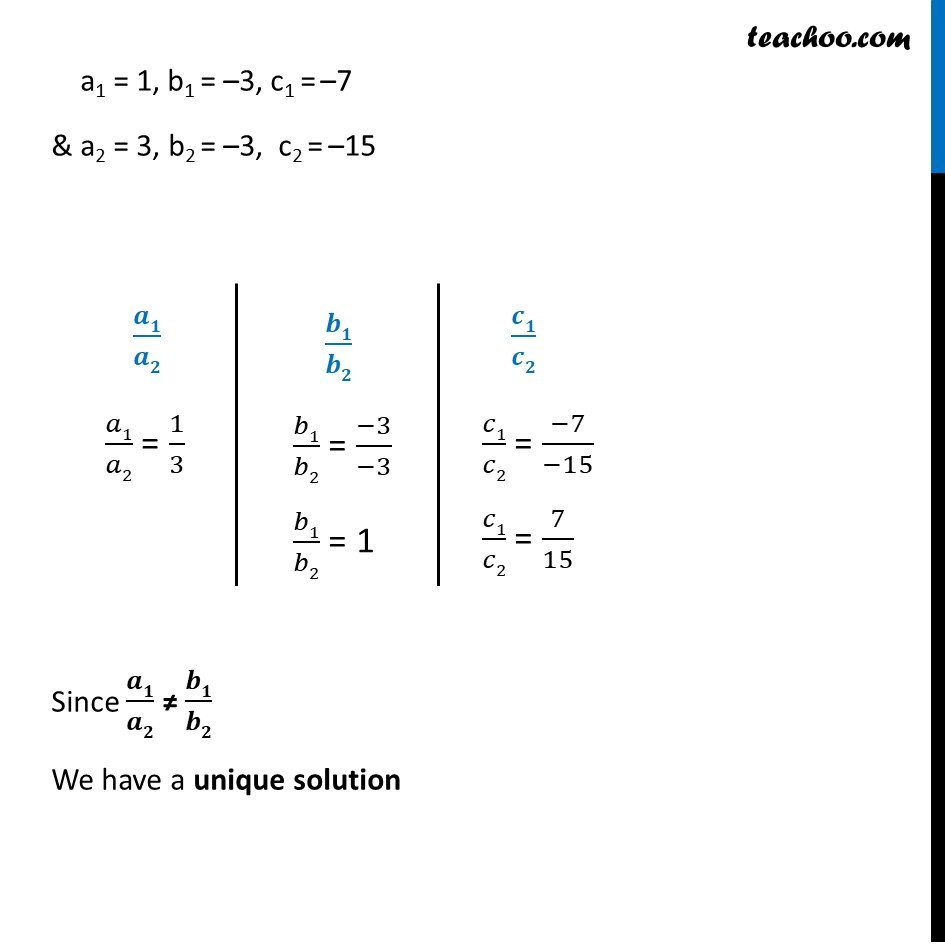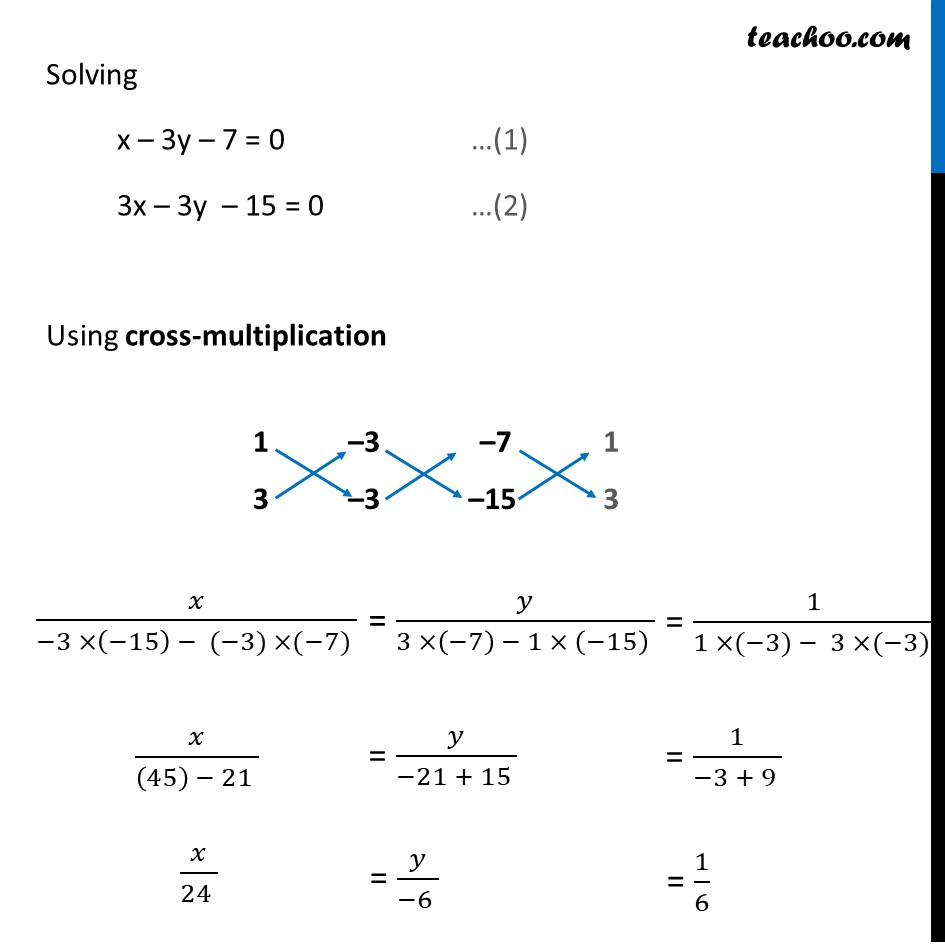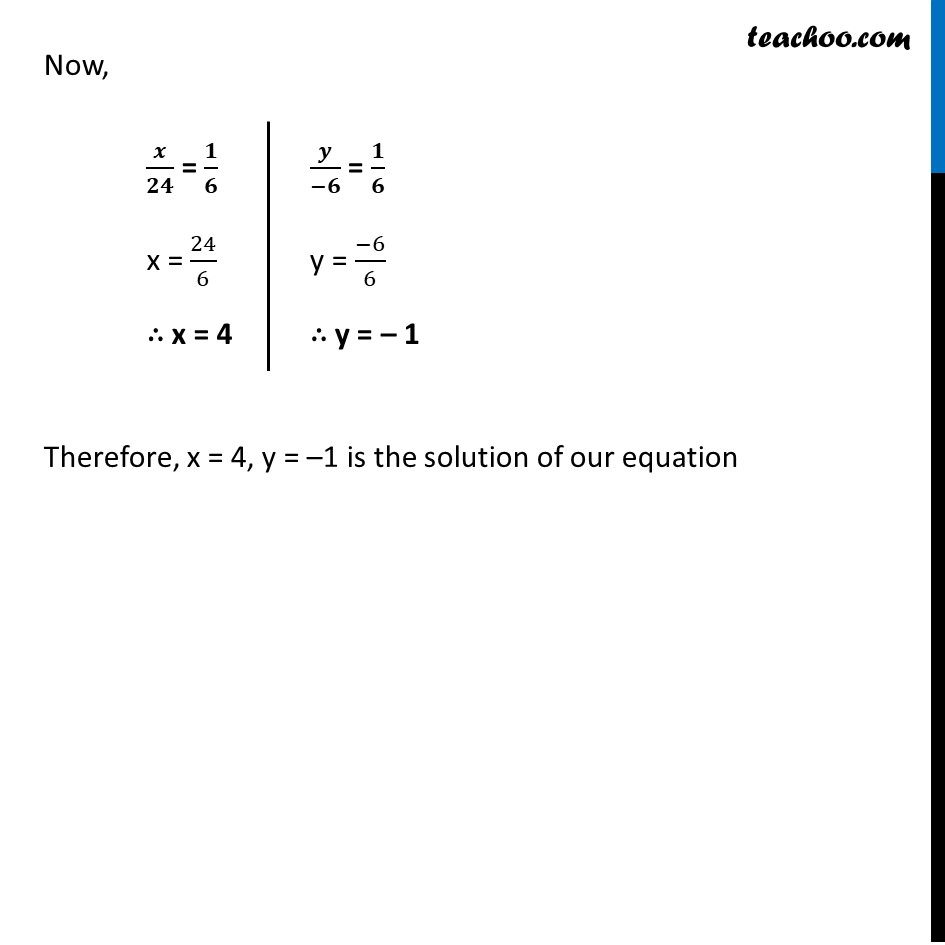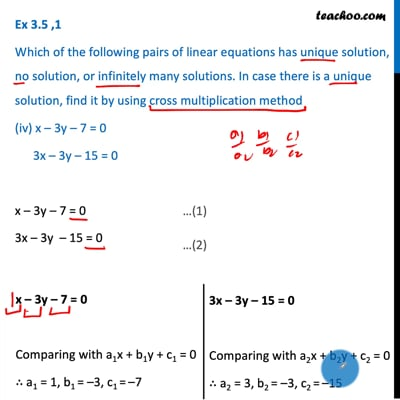This video is only available for Teachoo black users

Introducing your new favourite teacher - Teachoo Black, at only ₹83 per month

### Transcript

Ex 3.5 ,1 Which of the following pairs of linear equations has unique solution, no solution, or infinitely many solutions. In case there is a unique solution, find it by using cross multiplication method (iv) x – 3y – 7 = 0 3x – 3y – 15 = 0 x – 3y – 7 = 0 3x – 3y – 15 = 0 x – 3y – 7 = 0 Comparing with a1x + b1y + c1 = 0 ∴ a1 = 1, b1 = –3, c1 = –7 3x – 3y – 15 = 0 Comparing with a2x + b2y + c2 = 0 ∴ a2 = 3, b2 = –3, c2 = –15 a1 = 1, b1 = –3, c1 = –7 & a2 = 3, b2 = –3, c2 = –15 𝒂𝟏/𝒂𝟐 𝑎1/𝑎2 = 1/3 𝒃𝟏/𝒃𝟐 𝑏1/𝑏2 = (−3)/(−3) 𝑏1/𝑏2 = 1 𝒄𝟏/𝒄𝟐 𝑐1/𝑐2 = (−7)/(−15) 𝑐1/𝑐2 = 7/15 Since 𝒂𝟏/𝒂𝟐 ≠ 𝒃𝟏/𝒃𝟐 We have a unique solution Solving x – 3y – 7 = 0 …(1) 3x – 3y – 15 = 0 …(2) Using cross-multiplication 𝑥/(−3 ×(−15) − (−3) ×(−7) ) = 𝑦/(3 ×(−7) − 1 × (−15) ) = 1/(1 ×(−3) − 3 ×(−3) ) 𝑥/((45) − 21 ) = 𝑦/(−21 + 15 ) = 1/(−3 + 9 ) 𝑥/(24 ) = 𝑦/(−6 ) = 1/6 Now, 𝒙/𝟐𝟒 = 𝟏/𝟔 x = 24/6 ∴ x = 4 𝒚/(−𝟔) = 𝟏/𝟔 y = (−6)/6 ∴ y = – 1 Therefore, x = 4, y = –1 is the solution of our equation